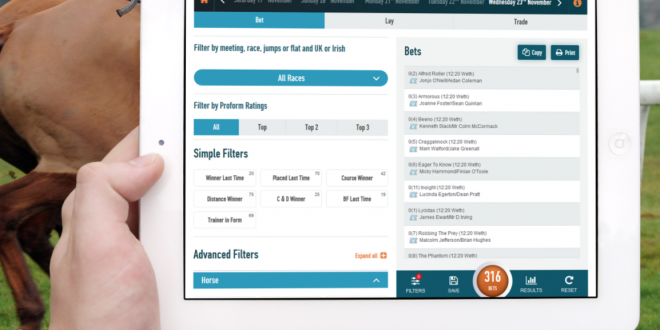Breaking News# Sports Betting: Converting Betting Odds to Implied Probabilities

One of the most fundamental parts of successful online sports betting practice is to understand how betting odds work. In other words, you need to understand the math behind each bet.

Thus, let’s consider the betting odds offered by a bookmaker as the probability that a specific team or individual will win the match.

Probability Theory: What is a probability?

According to Wikipedia.com, a probability is a “numerical description of how likely an event is to occur or how likely it is that a proposition is true.”

As a result, the higher the number that indicates whether a specific player or team will win a match, the higher the probability that this individual or team will win the game.

Finally, for the sake of completeness, probability theory is defined as the “branch of mathematics concerned with probability.”

The Types of betting odds

It is vital to note at this juncture that, you must sign up with a bona fide sportsbook to ensure that the betting odds calculation is fair and equitable. Otherwise, you stand to lose the total value of your bankroll. Thus, it is a good idea to look at an online sports betting review site like Silentbet.com  to find a legitimate sportsbook to ensure that you get a fair deal when betting on sporting events.

Before we look at how to convert betting odds into implied probabilities, let’s look at the three different types of betting odds, as this information is relevant to the probability calculation.

Decimal odds

Succinctly stated, decimal odds indicate the amount that you can win for every \$1 (USD) that you risk securing a bet. Therefore, if the odds of Player 1 winning a tennis match are 5.00, then the monies earned are five times the amount wagered. So, if you bet \$100 on Player 1 to win the game, you will win \$500.

Fractional odds

These odds are also known as British or traditional odds and are written as a fraction like 5/1 or as a ratio like five-to-one. Again, if we look at our example, if you wager \$50 on Player 1 winning the tennis match and the odds are 5/1, you will win \$250.

American odds

American odds, or Moneyline odds, are indicated by a + and – sign, accompanied by a number. The – sign indicates the favorite to win the match while the + sign indicates the underdog.

Converting betting odds into probabilities

Investopedia.com states that the formula or rule to calculate any types of odds into an implied probability is as follows:

The implied probability of an outcome = Stake (Amount Wagered)/Total Payout.

For example, if we use fractional odds and assume the odds of Player 1 winning the tennis match highlighted above are 5/15. The Stake is 5, and the total Payout is 15. Thus, in this scenario, the implied probability = 5/15 x 100 to get the percentage. And the odds of Player 1 winning the match is 33.3%

The probability of Player 1 winning the match with the decimal odds of 2.20 is calculated as 1 / 2.2 x 100 shows that there is a 45.45% of Player 1 winning the match.